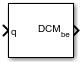Main Content

# Quaternions to Direction Cosine Matrix

Convert quaternion vector to direction cosine matrix

• Library:
• Aerospace Blockset / Utilities / Axes Transformations

•## Description

The Quaternions to Direction Cosine Matrix block transforms a four-element unit quaternion vector (q0, q1, q2, q3) into a 3-by-3 direction cosine matrix (DCM). The outputted DCM performs the coordinate transformation of a vector in inertial axes to a vector in body axes. Aerospace Blockset™ uses quaternions that are defined using the scalar-first convention. For more information, see Algorithms.

## Ports

### Input

expand all

Quaternion, specified as a 4-by-1 vector.

Data Types: `double`

### Output

expand all

Direction cosine matrix, returned as a 3-by-3 matrix.

Data Types: `double`

## Algorithms

Using quaternion algebra, if a point P is subject to the rotation described by a quaternion q, it changes to P′ given by the following relationship:

`$\begin{array}{l}{P}^{\prime }=qP{q}^{c}\\ q={q}_{0}+i{q}_{1}+j{q}_{2}+k{q}_{3}\\ {q}^{c}={q}_{0}-i{q}_{1}-j{q}_{2}-k{q}_{3}\\ P=0+ix+jy+kz\end{array}$`

Expanding P′ and collecting terms in x, y, and z gives the following for P′ in terms of P in the vector quaternion format:

`${P}^{\prime }=\left[\begin{array}{c}0\\ {x}^{\prime }\\ {y}^{\prime }\\ {z}^{\prime }\end{array}\right]=\left[\begin{array}{c}0\\ \left({q}_{0}^{2}+{q}_{1}^{2}-{q}_{2}^{2}-{q}_{3}^{2}\right)x+2\left({q}_{1}{q}_{2}-{q}_{0}{q}_{3}\right)y+2\left({q}_{1}{q}_{3}+{q}_{0}{q}_{2}\right)z\\ 2\left({q}_{0}{q}_{3}+{q}_{1}{q}_{2}\right)x+\left({q}_{0}^{2}-{q}_{1}^{2}+{q}_{2}^{2}-{q}_{3}^{2}\right)y+2\left({q}_{2}{q}_{3}-{q}_{0}{q}_{1}\right)z\\ 2\left({q}_{1}{q}_{3}-{q}_{0}{q}_{2}\right)x+2\left({q}_{0}{q}_{1}+{q}_{2}{q}_{3}\right)y+\left({q}_{0}^{2}-{q}_{1}^{2}-{q}_{2}^{2}+{q}_{3}^{2}\right)z\end{array}\right]$`

Since individual terms in P′ are linear combinations of terms in x, y, and z, a matrix relationship to rotate the vector (x, y, z) to (x′, y′, z′) can be extracted from the preceding. This matrix rotates a vector in inertial axes, and hence is transposed to generate the DCM that performs the coordinate transformation of a vector in inertial axes into body axes.

`$DCM=\left[\begin{array}{lll}\left({q}_{0}^{2}+{q}_{1}^{2}-{q}_{2}^{2}-{q}_{3}^{2}\right)\hfill & 2\left({q}_{1}{q}_{2}+{q}_{0}{q}_{3}\right)\hfill & 2\left({q}_{1}{q}_{3}-{q}_{0}{q}_{2}\right)\hfill \\ 2\left({q}_{1}{q}_{2}-{q}_{0}{q}_{3}\right)\hfill & \left({q}_{0}^{2}-{q}_{1}^{2}+{q}_{2}^{2}-{q}_{3}^{2}\right)\hfill & 2\left({q}_{2}{q}_{3}+{q}_{0}{q}_{1}\right)\hfill \\ 2\left({q}_{1}{q}_{3}+{q}_{0}{q}_{2}\right)\hfill & 2\left({q}_{2}{q}_{3}-{q}_{0}{q}_{1}\right)\hfill & \left({q}_{0}^{2}-{q}_{1}^{2}-{q}_{2}^{2}+{q}_{3}^{2}\right)\hfill \end{array}\right]$`

## Extended Capabilities

### C/C++ Code GenerationGenerate C and C++ code using Simulink® Coder™.

Introduced before R2006a

## SupportGet trial now